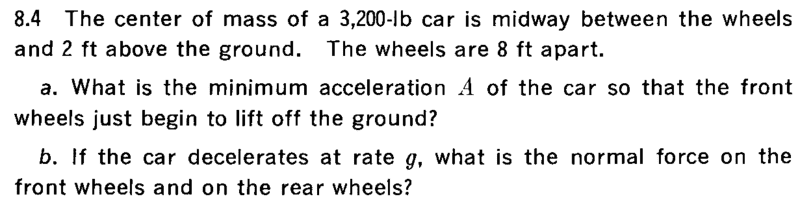# Minimum acceleration of a car at which front wheels leave the ground

## Homework Statement## The Attempt at a Solution

I assume that the wheels are 8ft apart in both x and y direction.
Let's denote the length and width of the car by 2l = 8 ft.
The torque about an axis connected the contact points of rear wheels :
##\vec \tau = [\{l( \hat x + \hat y) + h \hat z\}\times m( -g \hat z - A \hat x)]
\\ = m[ (lg- hA)\hat y + ml ( -g \hat x + A \hat z )##
Since the car is rotating along z axis, I take component of torque along y-axis to be 0 and this gives me A = lg/h.
But how to make component of torque along x-axis 0?

## Answers and Replies

.Scott
Homework Helper
When the front wheels leave the ground, the car will be rotating about the lateral axis - Based on your 1st statement in section 3, I believe that is your y axis, not z.
Assuming the car has no suspension, the front end will begin to rise just as the acceleration vector passes through the center of gravity.
So we need to look at the X and Z axis. Taking the rear wheel contact point at x,z = 0,0 and using units of feet, the center of gravity is at 4,2. Using acceleration units of "G", your acceleration will be x,z = x,1G.

So make them line up.

It does seem you have attempted a solution for the deceleration problem.

TSny
Homework Helper
Gold Member
The torque about an axis connected the contact points of rear wheels :
##\vec \tau = [\{l( \hat x + \hat y) + h \hat z\}\times m( -g \hat z - A \hat x)]
\\ = m[ (lg- hA)\hat y + ml ( -g \hat x + A \hat z )##
If you are using the definition ##\vec{\tau} = \vec r \times \vec F##, then ##\vec r## is the position vector of the force as measured from your chosen point of origin. It appears that you are taking the origin at the point of contact of one of the rear wheels with the ground. It would be nice to clearly state the location of the origin.

It appears that you have chosen your frame of reference to be moving with the car, so one of your forces is the fictitious force associated with the acceleration. It would be nice to state your choice of reference frame.

Also, make sure you have included the torques due to all of the forces. Are there forces acting at the points of contact of the rear wheels with the ground?

It is not necessary to assume that the distance between the rear wheels is the same as the distance between the two axles.

EDIT: You can think of the car as trying to rotate about a fixed axis in the accelerating frame. For an object rotating about a fixed axis, you can calculate the torques as "force times lever arm" and then you don't need to consider torques about a point of origin. So, you don't need to construct position vectors relative to a point. Instead, you just need to consider lever arms relative to an axis. The answer falls out very quickly this way. But, it's also a nice exercise to work it out using torques relative to an origin (point).

Last edited:
CWatters
Science Advisor
Homework Helper
Gold Member
I would suggest you make a free body diagram.